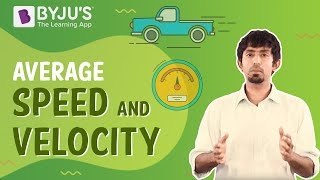# Acceleration Questions

The rate at which velocity varies with time is known as acceleration. Acceleration is a vector quantity because it has both a magnitude and direction. Acceleration is expressed in metres per second squared. Since acceleration is defined as the rate of change of velocity, the following are the different types of acceleration:

## Important Questions on Acceleration

1) A rope held by a monkey will not break when a mass of 30 kg is suspended to it, but if the mass exceeds 30 kg, it will break. If the mass of the monkey is 25 kg, find the minimum acceleration with which the monkey can climb up along the rope?

(g = 10m/s2)

a) 15m/s2

b) 1m/s2

c) 2m/s2

d) 5.5m/s2

Correct Option: (c)

Explanation: When the monkey climbs up the rope with acceleration a,

T = m(g+a)

Mg = mg+ma

30×10=25×10+25a

a = 2m/s2.

2) What does the displacement-time graph of a uniformly accelerated motion look like?

a) Hyperbola

b) Parabola

c) Straight Line

d) Ellipse

Correct Option: (b)

Explanation: The acceleration remains constant in a uniformly accelerated motion. Therefore, using the equation, s = ut + (1/2) at2 for displacement, it becomes the equation of a parabola.

3) What will be the acceleration of a ball if it falls from a building and covers a distance of 6m in 12sec.?

a) 0.11 m/s2

b) 0.22 m/s2

c) 9.81 m/s2

d) 0.08 m/s2

Correct Option: (d)

Explanation: Let us assume that the ball falls with initial velocity zero, now, applying the second equation of motion, s = ut + (1/2)at2 where t = 12s and s = 6m we get a = 0.08 m/s2.

4) Negative acceleration is also known as_____.

a) Relaxation

b) Deceleration

c) Elevation

d) Escalation

Correct Option: (a)

Explanation: The acceleration acting in the opposite direction of motion is known as retardation, and it is also termed as deceleration.

5) Which among the following is an accurate depiction for the deceleration when a car is travelling in a north direction and produces a deceleration of 80 m/s2 to stop?

a) Westwards, 80 m/s2

b) Southwards, 80 m/s2

c) Eastwards, 80 m/s2

d) Northwards, 80 m/s2

Correct Option: (b)

Explanation: Since the acceleration acting in the opposite direction of motion is known as deceleration, here, the deceleration has a magnitude of 80 m/s2 acting in the opposite direction to North. Hence the correct answer will be Southwards, 80 m/s2.

6) The velocity of a truck fluctuates with time as v = 6t3. What is the acceleration at t = 3?

a) 160

b) 156

c) 140

d) 162

Correct Option: (d)

Explanation: By differentiating the expression for velocity, the acceleration of a body can be calculated. Here v = 6t3. Hence, after differentiating, a becomes = dv/dt = 18t2. Putting t = 3, a = 162 units.

7) What is uniform acceleration?

An object is said to be in uniform acceleration when it is travelling in a straight line with an increase in velocity that takes place at equivalent intervals of time. One of the most common examples of uniform acceleration is a free fall of an object.

8) Define acceleration and state whether it’s a scalar or vector quantity.

The rate at which velocity variates with time is known as acceleration in terms of both speed and direction. If an object moving in a straight-line speeds up or slows down, it is said to be in accelerated motion. Acceleration is a vector quantity because it has both a magnitude as well as direction.

# 9) Find the value of instantaneous acceleration if the displacement is given by x=1+3t+4t2.

Given that,

x=1+3t+4t2

Now, differentiating the above equation to calculate the velocity,

x=1+3t+4t2

v = dx/dt ​= 0+3+8t

Now, again differentiating the velocity to calculate acceleration,

a = dv/dt ​= d2x/dt2​= 8m/s2

Hence, the instantaneous acceleration is 8 m/s2

10) It takes 6sec to touch the water surface when a stone is released freely into the river from a bridge.

(a) What is the height of the bridge from the water level?

(b) What is the distance covered by stone in the last second. Take g=9.8m/s2

Given :

Initial speed=u= 0 m/s

time = t = 6sec

(a)

height of bridge = distance travelled=h m

Using the second equation of motion:

s =h = ut+1/2at²

h =0xt +1/2 9.8 x 6 x6

h = 176.4m

∴The height of the bridge from the water level is 176.4m

(b)

Distance travelled in 5 sec

= (1/2×9.8x5x5)

= 122.5 m

Therefore, the distance travelled in the last second :

=Distance travelled in 6sec – distance travelled in 5 sec= 176.74 – 122.5 = 54.24m

∴The distance covered by stone in the last second is 54.24m

### Practice Questions

1) Define uniform and non-uniform acceleration.

2) What is uniform velocity?

3) What is the relationship between acceleration and velocity?

4) What is the acceleration due to gravity on Earth?

5) What are the different types of acceleration?

 Related Articles

## Suggested Videos

#### Motion Graphs | Velocity-Time Graph and Displacement-Time Graph#### Average Speed And Average Velocity#### Uniform Motion and Non Uniform Motion#### Understanding Uniform Circular Motion : Class 11-12